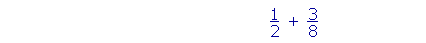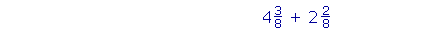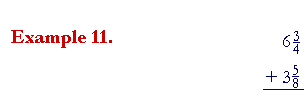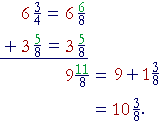S k i l l
i n
A R I T H M E T I C

Lesson 24

ADDING FRACTIONS
SUBTRACTING FRACTIONS

In this Lesson, we will answer the following:

1. How do we add or subtract fractions?
2. How do we add fractions that do not have the same denominator?
3. What number should we choose as the common denominator?
4. How do we add mixed numbers?

Section 2

5. How do we subtract a mixed number from a whole number?

 1. How do we add or subtract fractions? The units -- the denominators -- must be the same.  Add or subtract only the numerators.

 Example 1. 58 + 28 = 7 8 .

Just as

5 apples + 2 apples = 7 apples,

so

5 eighths + 2 eighths = 7 eighths.

To add anything, the units -- the names -- must be the same.  To add apples and oranges, you have to call them "piece of fruit"!

 In this problem, the unit is 18 .  We are adding eighths. (Lesson 20.)
 Example 2. 58 − 28 = 38 .

 2. How do we add or subtract fractions that do not have the same denominator?Make the denominators the same by changing to equivalent fractions. (Lesson 21.)
 3. What number should we choose as the common denominator? Choose a common multiple of the original denominators. Choose their lowest common multiple. (Lesson 22)

 Example 3. 12 + 38 .

Solution.  The lowest common multiple of 2 and 8, is 8 itself.  We will

 change 12 to a fraction whose denominator is 8.
 12 = 48 ,  because 4 is half of 8.

Therefore,

 12 + 38 = 48 + 38 = 78 .

In practice, it is necessary to write the common denominator only once:

 12 + 38 = 4 + 3   8 = 78 .

 Example 4. 45 + 2 15

Solution.  The LCM of 5 and 15  is 15.  Therefore,

 45 + 2 15 = 12  +  2    15 = 1415 .
 45 has been changed to 1215 by multiplying both terms by 3.
 2 15 has not been changed , because we are keeping the denominator 15.

 Example 5. 23 + 16 + 7 12

Solution.  The LCM of 3, 6, and 12  is 12.

 23 + 16 + 7 12 = 8 + 2 + 7     12
 23 + 16 + 7 12 = 1712
 23 + 16 + 7 12 =  1 5 12 .
 23 has been changed to 8 12 by multiplying both terms by 4.
 16 has been changed to 2 12 by multiplying both terms by 2.
 7 12 has not been changed, because we are keeping the denominator 12.
 The improper fraction 1712 has been changed to 1 5 12 by dividing 17 by 12.

"12 goes into 17 one (1) time with remainder 5."

 Example 6. 56 + 79

Solution.  The LCM of 6 and 9  is 18.

 56 + 79 = 15 + 14   18 = 2918 =  1 1118 .
 56 has been changed to 1518 by multiplying both terms by 3.
 79 has been changed to 1418 by multiplying both terms by 2.

 Example 7.    Add mentally 12 + 14 .
 Answer. 12 is how many 14 's?
 12 = 24 ,  because 2 is half of 4.

Therefore,

 12 + 14 = 34 .

The student should not have to write any problem in which one of

 the numbers is 12 .

For example,

 12 + 2 10 = 7 10
 because 12 = 5 10 .

Example 8.   In a recent exam, one eighth of the students got A, two fifths got B, and the rest got C.  What fraction got C?

Solution.   Let the whole number of students be represented by 1.  Then the question is:

 18 + 25 + ?  = 1 .

Now,

 18 + 25 = 5 + 16   40 = 2140 .
 The rest, the fraction that got C, is the complement of 2140 .
 It is 1940 .

 4. How do we add mixed numbers?Add the whole numbers and add the fractions.

 Example 9.    4 38 +  2 28 =  6 58 .

 Example 10.    3 25 +  1 45 =  4 65 .
 But 65 is improper, we must change it to a mixed number:
 46  5 = 1 15 .

Therefore,

 4 65 =  4 + 1 15 =  5 15 .Solution.  When the denominators are different, we may arrange the work vertically.

To add the fractions, the denominators must be the same.  The LCM

 of 4 and 8  is 8.  We will change 34 to 68 -- we will multiply both terms

by 2:We added  6 + 3 = 9. 68 + 58 = 11 8 = 1 38 .
 9 + 1 38 = 10 38 .

At this point, please "turn" the page and do some Problems.

or

Continue on to the next Section.

www.proyectosalonhogar.com